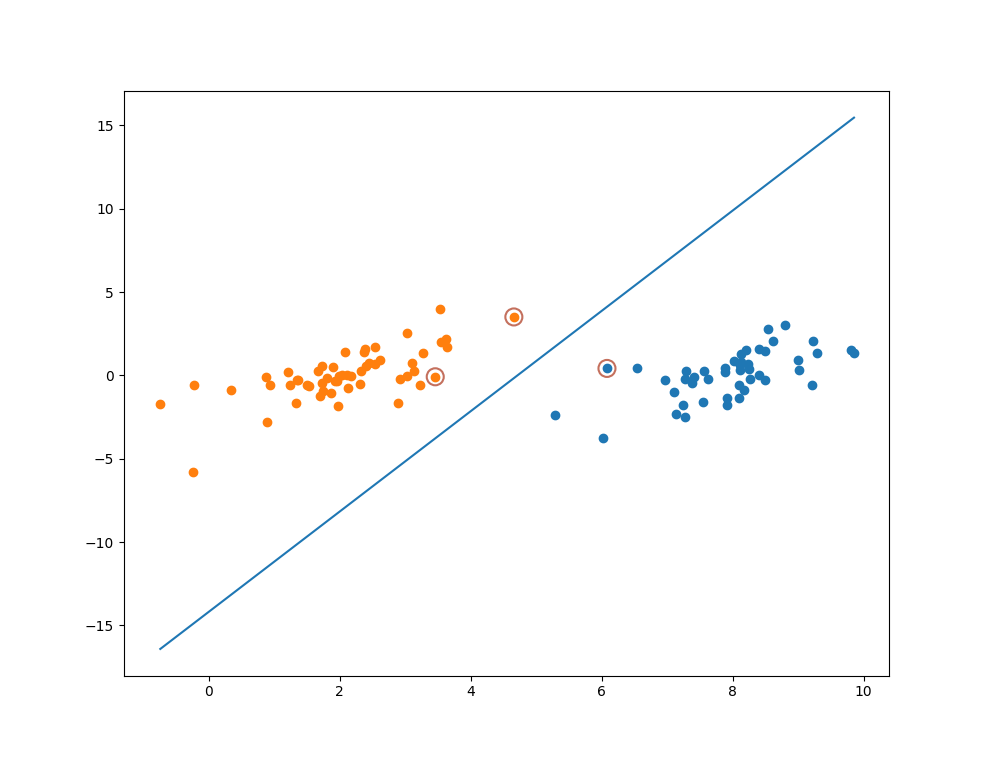## 坐标上升算法(Coordinate Ascent)## 例子1. 先固定，把看做的一元函数求最优值，可以简单的进行求导获取解析解:2. 在固定x2x2, 把ff看成x1x1的一元函数求最优值，得到x1x1的解析解:## SMO算法介绍

SMO的思想类似坐标上升算法，我们需要优化一系列的αα的值，我们每次选择尽量少的来优化，不断迭代直到函数收敛到最优值。subject to,## 获得没有修剪的原始解,的表达式代入得到:以及的值可以表示成:的表达式代入到中可以得到:得到最终的一阶导数表达式:## 对原始解进行修剪(如左图) 当时，线性限制条件可以写成:，根据的正负可以得到不同的上下界，因此统一表示成:

• 下界:• 上界:(如右图) 当时，限制条件可写成:, 上下界表示成:

• 下界:• 上界:OK， 这样我们就知道如何将选取的一对进行优化更新了。

## 更新阈值b不在边界，即, 根据KKT条件可知相应的数据点为支持向量，满足, 两边同时乘上得到, 进而得到的值:, 可以得到bnew2b2new的表达式(推导同上):都有效的时候他们是相等的, 即## 简化版SMO算法实现

def load_data(filename):
dataset, labels = [], []
with open(filename, 'r') as f:
for line in f:
x, y, label = [float(i) for i in line.strip().split()]
dataset.append([x, y])
labels.append(label)
return dataset, labels
def clip(alpha, L, H):
''' 修建alpha的值到L和H之间.
'''
if alpha < L:
return L
elif alpha > H:
return H
else:
return alpha
def select_j(i, m):
''' 在m中随机选择除了i之外剩余的数
'''
l = list(range(m))
seq = l[: i] + l[i+1:]
return random.choice(seq)

def get_w(alphas, dataset, labels):
''' 通过已知数据点和拉格朗日乘子获得分割超平面参数w
'''
alphas, dataset, labels = np.array(alphas), np.array(dataset), np.array(labels)
yx = labels.reshape(1, -1).T*np.array([1, 1])*dataset
w = np.dot(yx.T, alphas)
return w.tolist()

def simple_smo(dataset, labels, C, max_iter):
''' 简化版SMO算法实现，未使用启发式方法对alpha对进行选择.
:param dataset: 所有特征数据向量
:param labels: 所有的数据标签
:param C: 软间隔常数, 0 <= alpha_i <= C
:param max_iter: 外层循环最大迭代次数
'''
dataset = np.array(dataset)
m, n = dataset.shape
labels = np.array(labels)
# 初始化参数
alphas = np.zeros(m)
b = 0
it = 0
def f(x):
"SVM分类器函数 y = w^Tx + b"
# Kernel function vector.
x = np.matrix(x).T
data = np.matrix(dataset)
ks = data*x
# Predictive value.
wx = np.matrix(alphas*labels)*ks
fx = wx + b
return fx[0, 0]

while it < max_iter:
pair_changed = 0
for i in range(m):
a_i, x_i, y_i = alphas[i], dataset[i], labels[i]
fx_i = f(x_i)
E_i = fx_i - y_i
j = select_j(i, m)
a_j, x_j, y_j = alphas[j], dataset[j], labels[j]
fx_j = f(x_j)
E_j = fx_j - y_j
K_ii, K_jj, K_ij = np.dot(x_i, x_i), np.dot(x_j, x_j), np.dot(x_i, x_j)
eta = K_ii + K_jj - 2*K_ij
if eta <= 0:
print('WARNING  eta <= 0')
continue
# 获取更新的alpha对
a_i_old, a_j_old = a_i, a_j
a_j_new = a_j_old + y_j*(E_i - E_j)/eta
# 对alpha进行修剪
if y_i != y_j:
L = max(0, a_j_old - a_i_old)
H = min(C, C + a_j_old - a_i_old)
else:
L = max(0, a_i_old + a_j_old - C)
H = min(C, a_j_old + a_i_old)
a_j_new = clip(a_j_new, L, H)
a_i_new = a_i_old + y_i*y_j*(a_j_old - a_j_new)
if abs(a_j_new - a_j_old) < 0.00001:
#print('WARNING   alpha_j not moving enough')
continue
alphas[i], alphas[j] = a_i_new, a_j_new
# 更新阈值b
b_i = -E_i - y_i*K_ii*(a_i_new - a_i_old) - y_j*K_ij*(a_j_new - a_j_old) + b
b_j = -E_j - y_i*K_ij*(a_i_new - a_i_old) - y_j*K_jj*(a_j_new - a_j_old) + b
if 0 < a_i_new < C:
b = b_i
elif 0 < a_j_new < C:
b = b_j
else:
b = (b_i + b_j)/2
pair_changed += 1
print('INFO   iteration:{}  i:{}  pair_changed:{}'.format(it, i, pair_changed))
if pair_changed == 0:
it += 1
else:
it = 0
print('iteration number: {}'.format(it))
return alphas, b

Ok, 下面我们就用训练数据对SVM进行优化, 并对最后优化的分割线以及数据点进行可视化

if '__main__' == __name__:
# 加载训练数据
dataset, labels = load_data('testSet.txt')
# 使用简化版SMO算法优化SVM
alphas, b = simple_smo(dataset, labels, 0.6, 40)
# 分类数据点
classified_pts = {'+1': [], '-1': []}
for point, label in zip(dataset, labels):
if label == 1.0:
classified_pts['+1'].append(point)
else:
classified_pts['-1'].append(point)
fig = plt.figure()
ax = fig.add_subplot(111)
# 绘制数据点
for label, pts in classified_pts.items():
pts = np.array(pts)
ax.scatter(pts[:, 0], pts[:, 1], label=label)
# 绘制分割线
w = get_w(alphas, dataset, labels)
x1, _ = max(dataset, key=lambda x: x)
x2, _ = min(dataset, key=lambda x: x)
a1, a2 = w
y1, y2 = (-b - a1*x1)/a2, (-b - a1*x2)/a2
ax.plot([x1, x2], [y1, y2])
# 绘制支持向量
for i, alpha in enumerate(alphas):
if abs(alpha) > 1e-3:
x, y = dataset[i]
ax.scatter([x], [y], s=150, c='none', alpha=0.7,
linewidth=1.5, edgecolor='#AB3319')
plt.show()

## 参考

1. 优化算法——坐标上升法
2. 支持向量机系列（5）——SMO算法解对偶问题
3. 《Machine Learning in Action》